NCERT Solution (Part - 2) - Accounting Ratios

# NCERT Solution (Part - 2) - Accounting Ratios - Notes | Study Additional Study Material for Commerce - Commerce

 1 Crore+ students have signed up on EduRev. Have you?

Page No.: 239

Numericals Questions:
Question 1: Current Ratio is 3.5:1 Working Capital is Rs 9,00,000. Calculate the amount of Current Assets and Current Liabilities.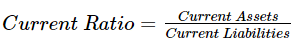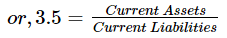or, Current Assets = 3.5 Current Liabilities (1)
Working Capital = Current Assets - Current Liabilities
Working Capital = 90,000
or, Current Assets - Current Liabilities = 90,000
or, 3.5 Current Liabilities - Current Liabilities = 90,000 (from 1)
or, 2.5 Current Liabilities = 90,000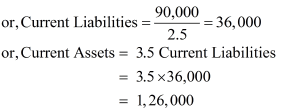Question 2: Shine Limited has a current ratio 4.5:1 and quick ratio 3:1; if the stock is 36,000, calculate current liabilities and current assets.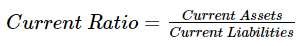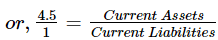or, 4.5 Current Liabilities = Current Assets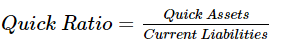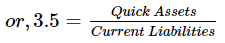or, 3 Current Liabilities = Quick Assets
Quick Assets = Current Assets- Inventory  = Current Assets - 36,000
Current Assets − Quick Assets = 36,000
or, 4.5 Current Liabilities − 3 Current Liabilities = 36,000
or, 1.5 Current Liabilities = 36,000
or, Current Liabilities = 24,000
Current Assets = 4.5 Current Liabilities
or, Current Assets = 4.5 x 24,000
= 1,08,000

Note: The solution given in the book is incorrect as it from the given figures Current Assets is ascertained to be Rs 1,08,000 and Current Liabilities Rs 24,000.

Question 3: Current liabilities of a company are Rs 75,000. If current ratio is 4:1 and liquid ratio is 1:1, calculate value of current assets, liquid assets and inventory
Answer: Current Ratio = Current Assets /Current Liabilities
or, 4 = Current Assets / 75,000
or, 4 × 75,000 = Current Assets
or, Current Assets = 3,00,000
Liquid Ratio = Liquid Assets / Current Liabilities
or, 1 = Liquid Assets / 75,000
Liquid Assets = 75,000
Inventory = Current Assets − Liquid Assets
= 3,00,000 − 75,000
= 2,25,000

Page No.: 240
Numericals Questions:

Question 1: Handa Ltd.has stock of Rs 20,000. Total liquid assets are Rs 1,00,000 and quick ratio is 2:1. Calculate current ratio.
Answer: Quick Ratio = Quick Assets / Current Liabilities
or, 2 =  1,00,000 / Current Liabilities
or, Current Liabilities =  1,00,000 / 2
= 50,000
Current Assets = Liquid Assets + Inventory
= 1,00,000 + 20,000
= 1,20,000
Current Ratio = Current Assets / Current Liabilities
=1,20,000/ 50,000
=2.4/1= 2.4:1

Question 2: Calculate debt equity ratio from the following information:

 Rs Total Assets 15,00,000 Current Liabilities 6,00,000 Total Debts 12,00,000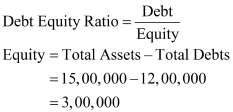Long Term Depts = Total Depts - Current Liabilities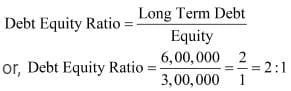Question 3: Calculate Current Ratio if:
Inventory is Rs 6,00,000; Liquid Assets Rs 24,00,000; Quick Ratio 2:1.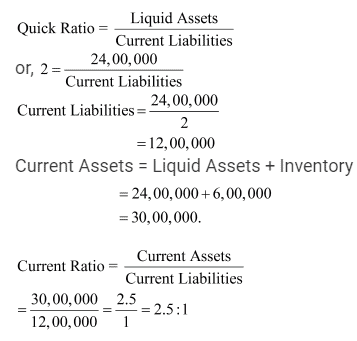Question 4: Compute Stock Turnover Ratio from the following information: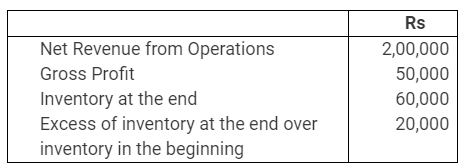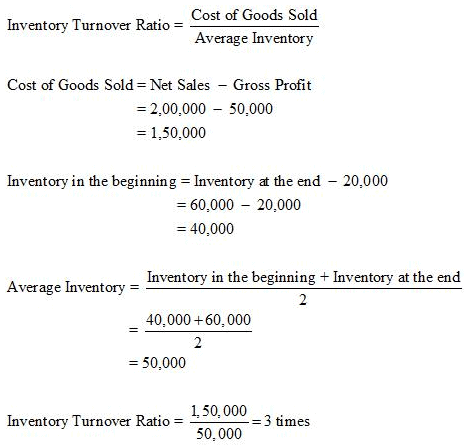Question 5: Calculate following ratios from the following information:
(i) Current ratio (ii) Acid test ratio (iii) Operating Ratio (iv) Gross Profit Ratio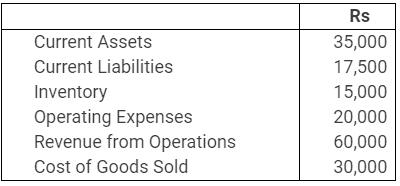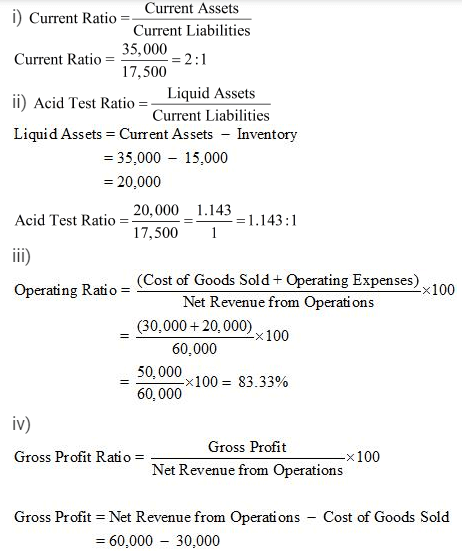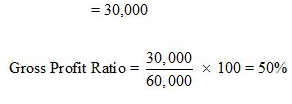Question 6: From the following information calculate:
(i) Gross Profit Ratio (ii) Inventory Turnover Ratio (iii) Current Ratio (iv) Liquid Ratio (v) Net Profit Ratio (vi) Working capital Ratio: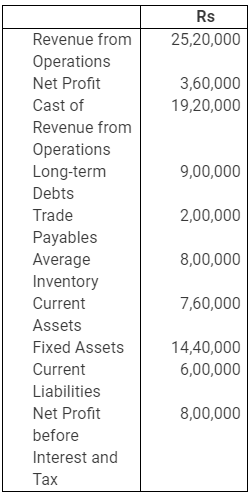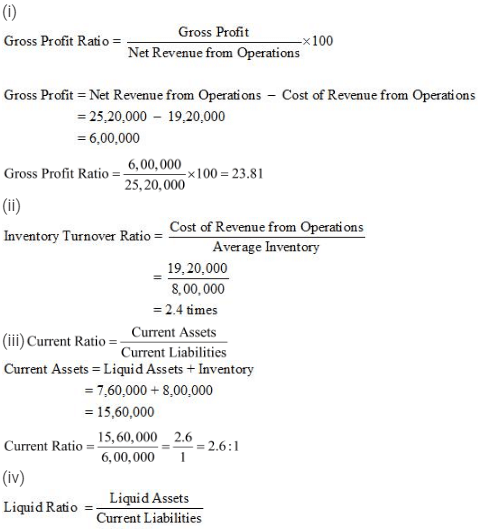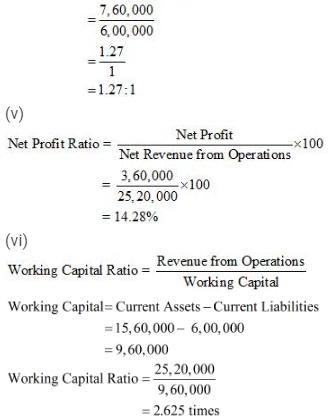NoteThere is a misprint in the question given in the textbook. The figure of Rs '760,000' represents the value of 'Liquid Assets' and not 'Current Assets'. The above solution has been worked out accordingly and the answer given as per the textbook is same as per the above solution.

Page No.: 241
Numericals Questions:
Question 1: Compute Gross Profit Ratio, Working Capital Turnover Ratio, Debt Equity Ratio and Proprietary Ratio from the following information: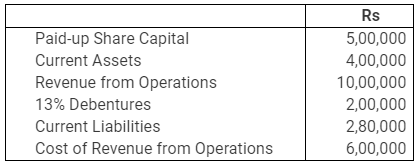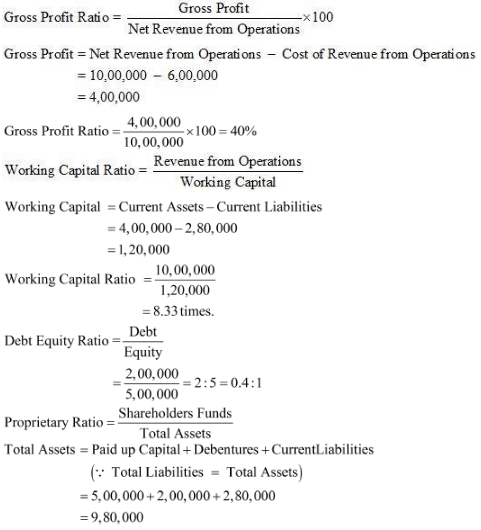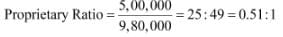Question 2: Calculate Inventory Turnover Ratio if:
Inventory in the beginning is Rs 76,250, Inventory at the end is 98,500, Gross Revenue from Operations is Rs 5,20,000, Return Inwards is Rs 20,000, Purchases is Rs 3,22,250.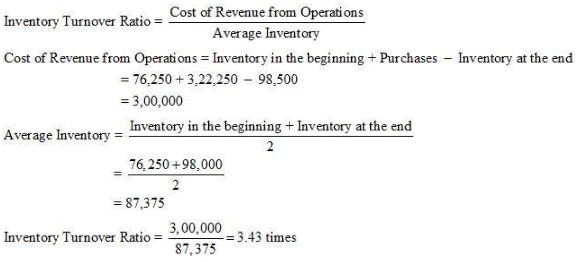The document NCERT Solution (Part - 2) - Accounting Ratios - Notes | Study Additional Study Material for Commerce - Commerce is a part of the Commerce Course Additional Study Material for Commerce.
All you need of Commerce at this link: Commerce

## Additional Study Material for Commerce

4 videos|168 docs

## Additional Study Material for Commerce

4 videos|168 docs

Track your progress, build streaks, highlight & save important lessons and more!

,

,

,

,

,

,

,

,

,

,

,

,

,

,

,

,

,

,

,

,

,

;